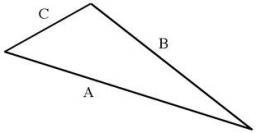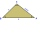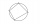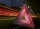# Short cut

Imagine that you are going to the friend. That path has a length 120 meters. Then turn doprava and go another 630 meters and you are at a friend's.

The question is how much the journey will be shorter if you go direct across the field?

Result

x =  108.7 m

#### Solution:

$a=120 \ \text{m} \ \\ b=630 \ \text{m} \ \\ \ \\ c_{0}=a+b=120+630=750 \ \text{m} \ \\ \ \\ c_{1}^2=a^2 + b^2 \ \\ \ \\ c_{1}=\sqrt{ a^2 + b^2 }=\sqrt{ 120^2 + 630^2 } \doteq 30 \ \sqrt{ 457 } \ \text{m} \doteq 641.3267 \ \text{m} \ \\ \ \\ x=c_{0}-c_{1}=750-641.3267 \doteq 108.6733 \doteq 108.7 \ \text{m}$

Try calculation via our triangle calculator.Our examples were largely sent or created by pupils and students themselves. Therefore, we would be pleased if you could send us any errors you found, spelling mistakes, or rephasing the example. Thank you!

Leave us a comment of this math problem and its solution (i.e. if it is still somewhat unclear...):Be the first to comment!Tips to related online calculators
Do you have a linear equation or system of equations and looking for its solution? Or do you have quadratic equation?
Pythagorean theorem is the base for the right triangle calculator.
Do you want to convert length units?

## Next similar math problems:

1. Interior anglesCalculate the interior angles of a triangle that are in the ratio 2: 3: 4.
2. Observation towerFrom the observation tower at a height of 105 m above sea level, the ship is aimed at a depth angle of 1° 49´. How far is the ship from the base of the tower?
3. The right triangleThe right triangle ABC has a leg a = 36 cm and an area S = 540 cm2. Calculate the length of the leg b and the median t2 to side b.
4. Concentric circles and chordIn a circle with a diameter d = 10 cm, a chord with a length of 6 cm is constructed. What radius have the concentric circle while touch this chord?
5. The trianglesThe triangles KLM and ABC are given, which are similar to each other. Calculate the lengths of the remaining sides of the triangle KLM, if the lengths of the sides are a = 7 b = 5.6 c = 4.9 k = 5
6. Side lengthsIn the triangle ABC, the height to the side a is 6cm. The height to side b is equal to 9 cm. Side "a" is 4 cm longer than side "b". Calculate the side lengths a, b.
7. In aIn a triangle, the aspect ratio a: c is 3: 2 and a: b 5: 4. The perimeter of the triangle is 74cm. Calculate the lengths of the individual sides.
8. The basesThe bases of the isosceles trapezoid ABCD have lengths of 10 cm and 6 cm. Its arms form an angle α = 50˚ with a longer base. Calculate the circumference and content of the ABCD trapezoid.
9. Viewing angleThe observer sees a straight fence 60 m long at a viewing angle of 30°. It is 102 m away from one end of the enclosure. How far is the observer from the other end of the enclosure?
10. Interior anglesIn a quadrilateral ABCD, whose vertices lie on some circle, the angle at vertex A is 58 degrees and the angle at vertex B is 134 degrees. Calculate the sizes of the remaining interior angles.
11. Triangular prismCalculate the surface of a regular triangular prism, the edges of the base are 6 cm long and the height of the prism is 15 cm.
12. The anglesThe angles in the triangle are in the ratio 12: 15: 9. Find the angles.
13. ProbabilityHow probable is a randomly selected three-digit number divisible by five or seven?
14. FlakesA circle was described on the square, and a semicircle above each side of the square was described. This created 4 "flakes". Which is bigger: the content of the central square or the content of four chips?
15. Annulus from triangleCalculate the content of the area bounded by a circle circumscribed and a circle inscribed by a triangle with sides a = 25mm, b = 29mm, c = 36mm
16. The angles ratioThe angles in the ABC triangle are in the ratio 1: 2: 3. find the sizes of the angles and determine what kind of a triangle it is.
17. The conicalThe conical candle has a base diameter of 20 cm and a side of 30 cm. How much dm ^ 3 of wax was needed to make it?